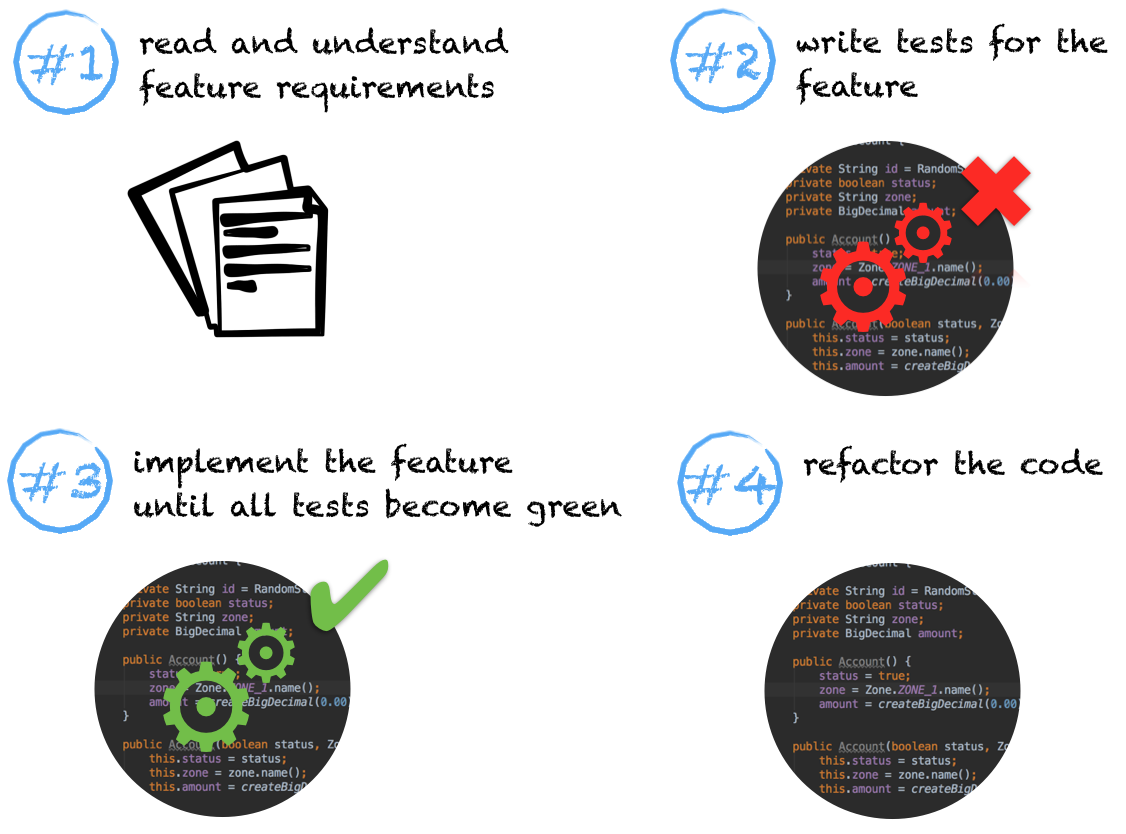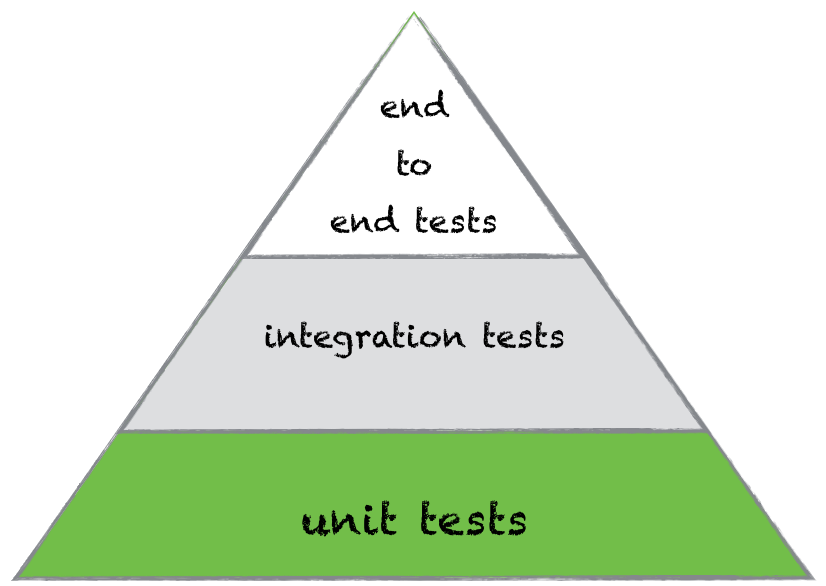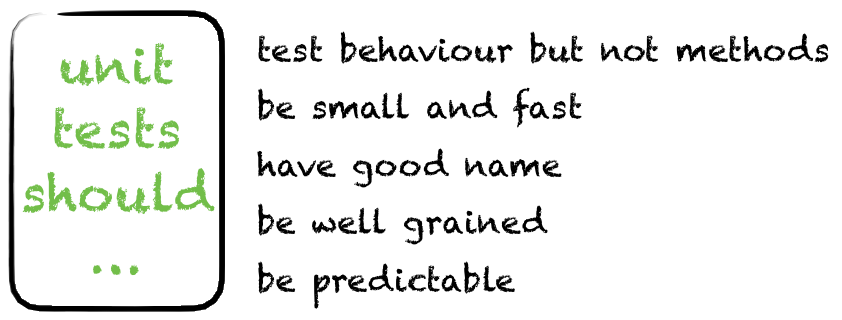Welcome to an introduction in Test Driven Development (TDD) series. We will talk about Java and JUnit in a context of TDD, but these are just tools. The main aim of the article is to give you a comprehensive understanding of TDD regardless of programming language and testing framework.

If you don’t use TDD in your project you are either lazy or you simply don’t know how TDD works. Excuses about lack of time don’t apply here.

In this post I’ll explain what is TDD and how it can be used in Java. Which place unit testing takes in TDD. What you have to cover with your unit tests. And finally, which principles you need to adhere in order to write good and effective unit tests.

If you have already know everything about TDD in Java, but you are interested in examples and tutorials, I recommend you to skip this part and continue with a (it will be published in one week after this one).

## What is TDD?

If somebody asks me to explain TDD in few words, I say TDD is a development of tests before a feature implementation. You can argue: it’s hard to test things which are not existing yet. And probably Kent Beck will give you a slap for this.

So how it’s possible? It can be described by following steps:

1. You read and understand requirements for a particular feature.
2. You develop set of tests which check the feature. All of the tests are red, due to absence of the feature implementation.
3. You develop the feature until all tests become green.
4. Refactoring of the code.TDD requires a different way of thinking, so in order to start working according to it you need to forget a way you developed a code before. This process is very hard. And it is even harder if you don’t know how to write unit tests. But it’s worth it.

Developing with TDD has valuable advantages:

1. You have a better understanding of a feature you implement.
2. You have robust indicators of a feature completeness.
3. A code is covered with tests and has less chances to be corrupted by fixes or new features.

A cost of these advantages is pretty high – inconvenience related to switching to a new development manner and time which you spend for developing of each new feature. It’s a price of quality.

So that’s how TDD works – write red unit tests, start implement a feature, make the tests green, perform refactor of the code.

## Place of unit tests in TDD

Since unit tests are the smallest elements in the test automation pyramid, TDD is based on them. With help of unit tests we can check business logic of any class. Writing of unit tests are easy if you know how to do this. So what you have to test with unit tests and how you need to do it? Do yon know answers on these questions? I’ll try to illustrate answers in a concise form.A unit test should be as small as possible. No-no don’t think about this as one test is for one method. For sure, this case is also possible. But as a rule, one unit test implies invocation of several methods. This is called testing of behaviour.

Let’s consider the Account class:

```public class Account {

private String id = RandomStringUtils.randomAlphanumeric(6);
private boolean status;
private String zone;
private BigDecimal amount;

public Account() {
status = true;
zone = Zone.ZONE_1.name();
amount = createBigDecimal(0.00);
}

public Account(boolean status, Zone zone, double amount) {
this.status = status;
this.zone = zone.name();
this.amount = createBigDecimal(amount);
}

public enum Zone {
ZONE_1, ZONE_2, ZONE_3
}

public static BigDecimal createBigDecimal(double total) {
return new BigDecimal(total).setScale(2, BigDecimal.ROUND_HALF_UP);
}

@Override
public String toString() {
StringBuilder sb = new StringBuilder();
sb.append("id: ").append(getId())
.append("\nstatus: ")
.append(getStatus())
.append("\nzone: ")
.append(getZone())
.append("\namount: ")
.append(getAmount());
return sb.toString();
}

public String getId() {
return id;
}

public boolean getStatus() {
return status;
}

public void setStatus(boolean status) {
this.status = status;
}

public String getZone() {
return zone;
}

public void setZone(String zone) {
this.zone = zone;
}

public BigDecimal getAmount() {
return amount;
}

public void setAmount(BigDecimal amount) {
if (amount.signum() < 0)
throw new IllegalArgumentException("The amount does not accept negative values");
this.amount = amount;
}
}
```

There are 4 getter methods in the class. Pay extra attention to them. If we create a separate unit test for each getter method, we get too many redundant lines of code. This situation can be handled with help of a behaviour testing. Imagine that we need to test a correctness of the object creation using one of its constructors. How to check that the object is created as expected? We need to check a value of each field. Hence getters can be used in this scenario.

Create small and fast unit tests, because they should be executed each time before commit to a git repository and new build to a server. You can consider an example with real numbers in order to understand importance of unit tests speed. Let’s assume a project has 1000 unit tests. Each of them takes 100ms. As a result run of all tests takes 1 minute and 40 seconds.
Actually 100ms is too long for an unit test, so you have to reduce a time of run by applying different rules and techniques, e.g. do not perform database connection in unit tests (by definition unit tests are isolated) or perform initialisations of expensive objects in the @Before block.

Choose good names for unit tests. A name of a test can be as long as you want, but it should represent what verification the test does. For example if I need to test a default constructor of the Account class, I’ll name it defaultConstructorTest. One more useful advice for choosing of a test’s name is writing of a test logic before you name the test. While you developing a test you understand what happens inside of it, as a result composing of name becomes easier.

Unit tests should be predictable. This is the most obvious requirement. I’ll explain it on example. In order to check operation of money transfer (with 5% fee) you have to know which amount you send and how much you get as output. This test scenario can be implemented as sending of 100 \$ and receiving of 95 \$.

And finally unit tests should be well-grained. When you put one logical scenario per test you can achieve an informative feedback from your tests. And in case of a single failure, you will not loose information about a rest of functionality.All these recommendations are aimed to improve the unit tests design. But there is one more thing you need to know – basics of test design techniques.

## Basics of test design techniques

Writing tests is impossible without a test data. For example when you are testing a money transferring system you set some amount in a send money field. The amount is a test data in this case. So which values you should to choose for testing? In order to answer on this question we need to go through the most popular test design techniques. General purpose of test design techniques is simplifying of composing a test data.

Firstly let’s pretend that we can send just positive, integer amount of money. Also we can not send more than 1000. That’s can be presented as:

0 < amount <= 1000;
amount in integer

All our test scenarios can be splited by two groups: positive & negative scenarios. The first one is for test data which is allowed by a system and leads to successful results. The second one is for so called “failure scenarios”, when we use inappropriate data for interaction with the system.

According to the classes of equivalence technique we can select single random integer number from the range (0; 1000]. Let it be 500. Since the system works for 500 it should work fine for all integer numbers from the range. So 500 is a valid value.
Also we can select invalid input from the range. It can be any number with floating point, for instance 125.50

Then we have to refer to the boundary testing technique. According to it we have to choose 2 valid values from the left and right sides of the range. In our case we take 1 as the lowest allowed positive integer and 1000 from the right side.
The next step is to choose 2 invalid values on boundaries. So it’s 0 and 1001.

So in the end we have 6 values which we need to use in the unit test:
(1, 500, 1000) – for positive scenarios
(0, 125.50, 1001) – for negative scenarios

## Summary

In this post I tried to explain all aspects of TDD and show how important unit tests are in the TDD. So I hope after such detailed and long bla-bla theory we can continue with practice. In my next article I’ll demonstrate how to implement test driven development in sample java application. Our aim is to develop tests before a functionality. We will do it step by step, starting from a documentation analysis and finishing with a code refactoring.

Be sure, that all test will be green =)